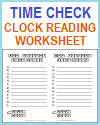Grade 1: Measurement and Data Worksheets
 Share the learning joy!

Grade 1 Math: Measurement and Data
 www.studenthandouts.com > First Grade > First Grade Math > Measurement and Data

CCSS.MATH.CONTENT.1.MD.A.1 - Order three objects by length; compare the lengths of two objects indirectly by using a third object.

CCSS.MATH.CONTENT.1.MD.A.2 - Express the length of an object as a whole number of length units, by laying multiple copies of a shorter object (the length unit) end to end; understand that the length measurement of an object is the number of same-size length units that span it with no gaps or overlaps. Limit to contexts where the object being measured is spanned by a whole number of length units with no gaps or overlaps.

CCSS.MATH.CONTENT.1.MD.B.3 - Tell and write time in hours and half-hours using analog and digital clocks.Time Check Clock-reading Handout
CCSS.MATH.CONTENT.1.MD.C.4 - Organize, represent, and interpret data with up to three categories; ask and answer questions about the total number of data points, how many in each category, and how many more or less are in one category than in another.